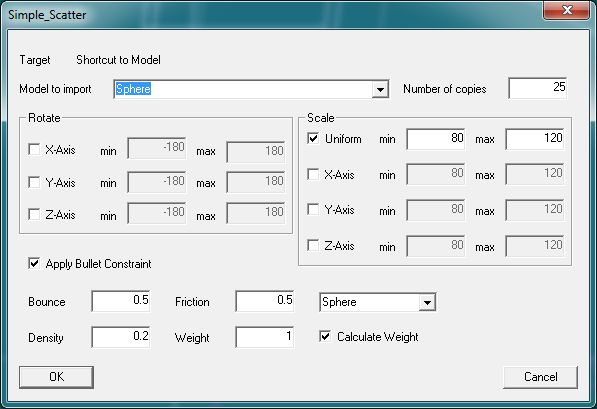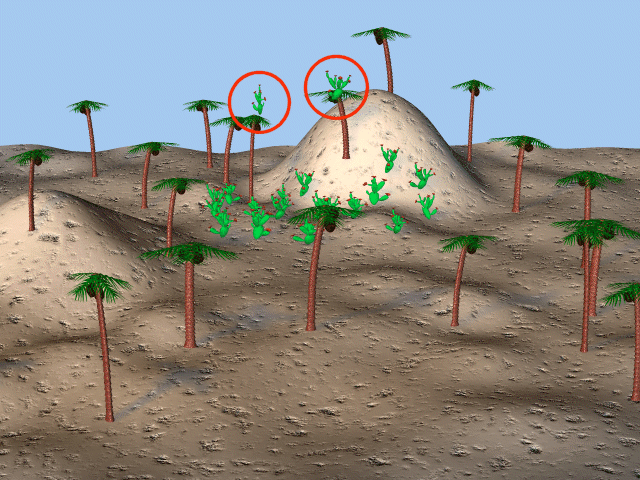# Plugin Simple Scatter

### Install

Nothing todo, it comes with the actual A:M distribution .
The plugin was originally only meant as faciltation of work for me and lays therefore by far no claim on completeness or accuracy.

### Description

With this plugin you can place a number of copies from one model on a target model. the imported models are randomly placed on the model or group where the plugin is started from .
To start the plugin select a model or one of his groups in a chor , then RMB->Plugins->Simple Scatter .
If the plugin isn't started from the frontview , it changes the view to frontview.Target the target model Model to import which model should be imported Number of copies how many we import this model . The imported models are randomly placed in the boundingbox from the selected target model/group. It can be that lesser models where imported, when the boundingboxes from the imported models are colliding while randomly placed Rotate If the desired checkbox is activated , then the model will be randomly rotated in the value range Scale If the desired checkbox is activated , then the model will be randomly scaled in the value range Apply Bullet Constraint Applies a Bullet Constraint to each instaced model, with the given properties. If "Calculate Weight" is checked , the value for "Weight" is ignored , instead the weight is calculated from the density and model volume . Makes sense, if models are scaled.